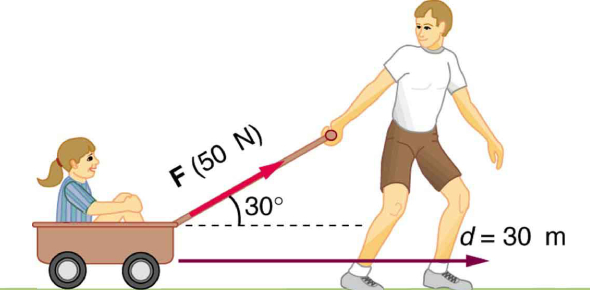# The Principles Of Work And Energy: Quiz!

10 Questions | Total Attempts: 2043SettingsIn everyday life, energy means the ability to do a specific work. The theory of work and energy are closely intertwined. Work is done on an object when an applied force moves it through a distance. This quiz has been developed for physics students and learners to test their basic knowledge about Work and Energy concepts.

• 1.
Mary pushes her cart across the floor with a force of 150N for a distance of 10m. What is the work done on the cart?
• A.

140 joules

• B.

200 joules

• C.

15 joules

• D.

1500 joules

• 2.
If Nancy pushes an object with twice the force for half the distance, she does
• A.

The same work

• B.

Twice the work

• C.

Half the work

• D.

Four times the work

• 3.
How much work is done on a 10kg rock that you lift 10m straight up?
• A.

500 joules

• B.

10 joules

• C.

1000 joules

• D.

1 joule

• 4.
Change in quality of environment that can be harmful and unpleasant for living things is called:
• A.

Energy

• B.

Pollution

• C.

Revolution

• D.

Transformation

• 5.
The energy possessed by a body due to its position is called its:
• A.

Heat energy

• B.

Kinetic energy

• C.

Potential energy

• D.

Chemical energy

• 6.
Work is being done only when a force is _______________ an object.
• A.

with

• B.

Moving

• C.

Next to

• D.

Stationary

• 7.
Work is done when a force moves an object a certain ______________
• A.

Distance

• B.

Way

• C.

Energy

• D.

Temperature

• 8.
When a force acting on a body displaces it in direction of a force then:
• A.

Uniform velocity occurs

• B.

Uniform speed occurs

• C.

Work is done

• D.

Equilibrium take place

• 9.
When a moving object has the energy of motion it has this type of energy.
• A.

Potential energy

• B.

High energy

• C.

Kinetic energy

• D.

Ransfer energy

• 10.
A moving car possesses:
• A.

Sound energy

• B.

Mechanical energy

• C.

Heat energy

• D.

Chemical energy

Related TopicsBack to top